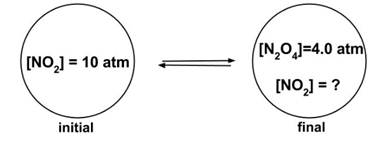# Enduring Understanding 6.A: Equilibrium Problems

• Sample Question 1: A chemical reaction will always be spontaneous (thermodynamically favored) under which conditions?
• a) Exothermic, with an increase in entropy
b) Exothermic, with a decrease in entropy
c) Endothermic, with a decrease in entropy
d) Endothermic, with an increase in entropy
• Answer: (a), Exothermic with an increase in entropy
• Thermodynamic favorability of a chemical reaction is determined by the Gibbs free energy,
• ΔG = ΔH - TΔS
• If ΔG < 0, the reaction is spontaneous.
• Therefore, if ΔH is negative (reaction is exothermic) and ΔS is positive (increase in entropy) then ΔG must always be negative.
• Sample Question 2: Consider the reaction X(g) + Y(g) ⇆ Z(g), with K = 10 at 25 °C
• Sample [X][Y] [Z]
10.5210
240.2510
34120
4115
• Which of the above samples are at equilibrium?
• a) Sample 1
b) Sample 2
c) Samples 1 and 2
d) Samples 1, 2, and 4
• Answer: (c), Samples 1 and 2
• The equilibrium constant is determined by K=[Z]/[X][Y]. If [Z]/[X][Y] = 10, the sample is at equilibrium. In both samples 1 and 2, [Z]/[X][Y] is 10, so they are both at equilibrium. In samples 3 and 4, [Z]/[X][Y] is 5, and the samples are not at equilibrium.
• Sample Question 3: Consider the reaction 2 NO2(g) ⇆ N2O4 (g). A rigid container is pressurized with 10 atm NO2, then allowed to come to equilibrium with a final concentration of 4.0 atm N2O4. What is Kp?
•a) 1
b) 2
c) 4
d) 10
• The problem can be solved as follows:
• Two equivalents NO2 are used to form 1 equivalent N2O4, so at 4.0 atm N2O4 the amount of NO2 remaining will be (10 - 2 x 4.0) or 2.0 atm.
• CompoundNO2N2O4
Initial pressure (atm)100
Final Pressure (atm)2.04.0
• So the equation for K is
• Kp = [N2O4] / [NO2]2 4.0 / (2.0)2 = 4.0 / 4.0 = 1.0

 Related Links: Chemistry Chemistry Quizzes AP Chemistry Notes Reversible Reactions

To link to this Equilibrium Problems page, copy the following code to your site: